Download Presentation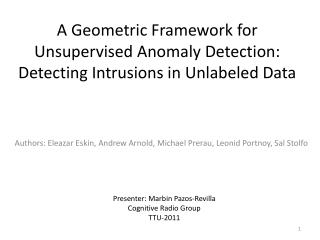A Geometric F ramework for Unsupervised A nomaly D etection: Detecting Intrusions in Unlabeled Data

# A Geometric F ramework for Unsupervised A nomaly D etection: Detecting Intrusions in Unlabeled Data - PowerPoint PPT Presentation

A Geometric F ramework for Unsupervised A nomaly D etection: Detecting Intrusions in Unlabeled Data. Authors: Eleazar E skin , Andrew Arnold, Michael Prerau , Leonid Portnoy , Sal Stolfo. Presenter: Marbin Pazos -Revilla Cognitive Radio Group TTU-2011. Motivation.I am the owner, or an agent authorized to act on behalf of the owner, of the copyrighted work described.
Download Presentation## A Geometric F ramework for Unsupervised A nomaly D etection: Detecting Intrusions in Unlabeled Data

An Image/Link below is provided (as is) to download presentation

Download Policy: Content on the Website is provided to you AS IS for your information and personal use and may not be sold / licensed / shared on other websites without getting consent from its author.While downloading, if for some reason you are not able to download a presentation, the publisher may have deleted the file from their server.

- - - - - - - - - - - - - - - - - - - - - - - - - - E N D - - - - - - - - - - - - - - - - - - - - - - - - - -
Presentation Transcript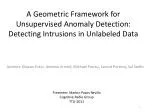### A Geometric Framework for Unsupervised Anomaly Detection: Detecting Intrusions in Unlabeled Data

Authors: EleazarEskin, Andrew Arnold, Michael Prerau, Leonid Portnoy, Sal Stolfo

Presenter: MarbinPazos-Revilla

TTU-2011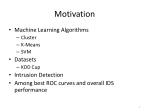Motivation
• Machine Learning Algorithms
• Cluster
• K-Means
• SVM
• Datasets
• KDD Cup
• Intrusion Detection
• Among best ROC curves and overall IDS performance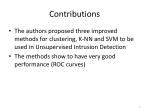Contributions
• The authors proposed three improved methods for clustering, K-NN and SVM to be used in Unsupervised Intrusion Detection
• The methods show to have very good performance (ROC curves)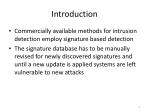Introduction
• Commercially available methods for intrusion detection employ signature based detection
• The signature database has to be manually revised for newly discovered signatures and until a new update is applied systems are left vulnerable to new attacks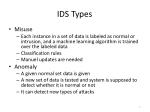IDS Types
• Misuse
• Each instance in a set of data is labeled as normal or intrusion, and a machine learning algorithm is trained over the labeled data
• Classification rules
• Manuel updates are needed
• Anomaly
• A given normal set data is given
• A new set of data is tested and system is supposed to detect whether it is normal or not
• It can detect new types of attacks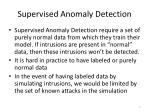Supervised Anomaly Detection
• Supervised Anomaly Detection require a set of purely normal data from which they train their model. If intrusions are present in “normal” data, then these intrusions won’t be detected.
• It is hard in practice to have labeled or purely normal data
• In the event of having labeled data by simulating intrusions, we would be limited by the set of known attacks in the simulation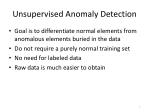Unsupervised Anomaly Detection
• Goal is to differentiate normal elements from anomalous elements buried in the data
• Do not require a purely normal training set
• No need for labeled data
• Raw data is much easier to obtain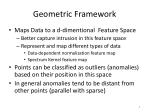Geometric Framework
• Maps Data to a d-dimentional Feature Space
• Better capture intrusion in this feature space
• Represent and map different types of data
• Data-dependent normalization feature map
• Spectrum Kernel feature map
• Points can be classified as outliers (anomalies) based on their position in this space
• In general anomalies tend to be distant from other points (parallel with sparse)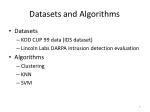Datasets and Algorithms
• Datasets
• KDD CUP 99 data (IDS dataset)
• Lincoln Labs DARPA intrusion detection evaluation
• Algorithms
• Clustering
• KNN
• SVM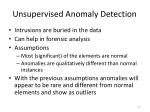Unsupervised Anomaly Detection
• Intrusions are buried in the data
• Can help in forensic analysis
• Assumptions
• Most (significant) of the elements are normal
• Anomalies are qualitatively different than normal instances
• With the previous assumptions anomalies will appear to be rare and different from normal elements and show as outliersGeometric Framework for Unsupervised Anomaly Detection
• Mapping records from audit stream to a feature space
• The distance between two elements in the feature space then becomes

orIn many cases is difficult to map data instances to a feature space and calculate distances

• High Dimentionality of the feature space (memory considerations)
• Explicit map might be difficult to determine
• We can define a kernel function to compute these dot products in the feature space (Hilbert)

Then we could get distances by using Kernel functionsRadial Basis Kernel Function

Defined over input spaces which are vector spaces

• Using Convolution kernels we can then use arbitrary input spaces.
• The author suggests the use of convolution kernels to avoid converting audit data into a vector inDetecting Outliers
• Detecting points that are distant from other points or in relatively sparse regions of the feature spaceCluster-based Estimation
• Count the number of points within a sphere of radius w around the point
• Sort clusters based on size
• The points in the small clusters are labeled anomalousCluster-based Estimation
• Any points x1,x2 are considered near if their distance is less than or equal to
• Define N(x) to be the number of points that are within w of point x
• Since we have to compute the pairwise distance among points the computation of N(x) for all points has complexity
• We are interested in the outliersTo reduce computation, an approximation can be done via fixed width clustering

• The first point is the center of the first cluster
• For every subsequent point, if it is within w of a cluster center, it is added to that cluster
• Otherwise it becomes the center of a new cluster
• Points may be added to several clusters
• Complexity with c number of clusters and n number of data points
• A threshold on n is used to find outliersK-Nearest Neighbor

• Find points that lie in a sparse region of the feature space by computing the distances to the k-nearest neighbors of the point
• Dense regions will have many points near them and will have a small k-NN score
• If k exceeds the frequency of any given attack and the images of the attack elements are far from the images of the normal elements, then the k-NN score can be used to detect attacksK-NN is computationally expensive

• Since we’re interested in only the k-nearest points to a given point we can reduce the computational cost by using canopy clustering
• Canopy Clustering is used to reduce the space into smaller subsets avoiding the need to check every data pointModified Canopy Clustering
• Cluster data with fixed-width approach with the variation of placing each element in only one cluster
• For each two points x1,x2 in a cluster
• And in all casesLet C be the set of clusters (initially containing all clusters in the data)

• At any step, we have a set of points which are potentially among the k-nearest neighbor points. This set is denoted as P.
• We also have a set of points that are in fact among the k-nearest points. This set is denotes as K.
• Initially K and P are emptyPre-compute the distance from x to each cluster.

• For the cluster with center closest to x we remove it from C and add all its points to P. Called Opening the Cluster
• We can use the lower bound on distance given by
• For each point xi in P we compare distances to other points in P
• If this distance is <dminwe can guarantee that xi is closer to point x than all the points in the clusters in CIn this case we remove xi from P and add it to K

• If distance is >dminthen we open the closest cluster and add all the points to P and remove that cluster from C
• Every time we remove a cluster from Cdmin will increase
• Once K has k elements we terminateComputation is spent checking distance between points in D to the cluster centers, which is more efficient than computing pairwise distances among all points

• Choice of w effects only the efficiency, not the K-NN score
• Intuitively we want to choose a w that splits the data into reasonably sized clustersOne Class SVM
• Map feature space into a second feature space with a radial basis kernel Standard SVM requires supervised learning algorithms (it requires labeled data)• Attempts to separate the entire set of data from the origin with maximal margin
• Classes will be labeled as +1 and -1The hyperplane is estimated by the hyperplane’s normal vector in the feature space w and offset from the origin

Decision functionFeature Space
• Data Sets
• Network Records with 41 features and 4,900,00 instances (KDD Cup 1999 Data)
• System Call Traces (process) from 5 weeks from the Basic Security Module of the MIT Lincoln Labs IDS Evaluation created on 1999